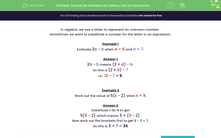# Substitute Numbers for Letters into an Expression

In this worksheet, students must substitute numbers in place of letters to evaluate the given expressions.Key stage:  KS 3

Curriculum topic:   Algebra

Curriculum subtopic:   Substitute Numerical Values for Formulae/Expressions

Difficulty level:#### Worksheet Overview

In algebra, we use a letter to represent an unknown number.

Sometimes we want to substitute a number for the letter in an expression.

Example 1

Evaluate 2a - b when a = 6 and b = 7.

2a - b means (2 × a) - b

So this is (2 × 6) - 7

i.e.  12 - 7 = 5.

Example 2

Work out the value of 5(x - 2) when x = 9.

Substitute x for 9 to get

5(9 - 2) which means 5 × (9 - 2).

Now work out the brackets first to get 9 - 2 = 7.

So this is 5 × 7 = 35

### What is EdPlace?

We're your National Curriculum aligned online education content provider helping each child succeed in English, maths and science from year 1 to GCSE. With an EdPlace account you’ll be able to track and measure progress, helping each child achieve their best. We build confidence and attainment by personalising each child’s learning at a level that suits them.

Get started